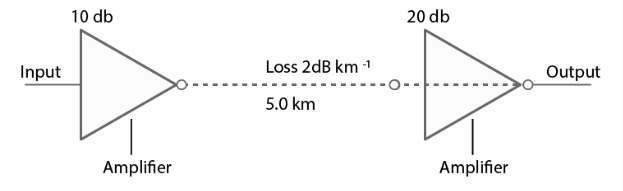Deepak Scored 45->99%ile with Bounce Back Crack Course. You can do it too!

# Figure 15.3 shows a communication system.

Question:

Figure 15.3 shows a communication system. What is the output power when the input signal is of 1.01mW? (gain in dB = 10 log10 (Po /Pi ).Solution:

Loss suffered in the path of transmission = 2 dB/km

Distance travelled by the signal = 5 km

Total loss suffered = -10 dB

Totla amplifier gain = 30 dB

Overall gain in signal = 20 dB

Gain in dB = 2

Input power = 1.01 mW

Po = 1.01 × 100

Output power is 101 mW.